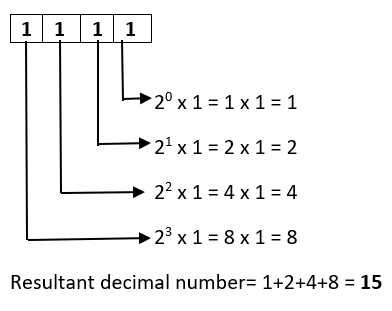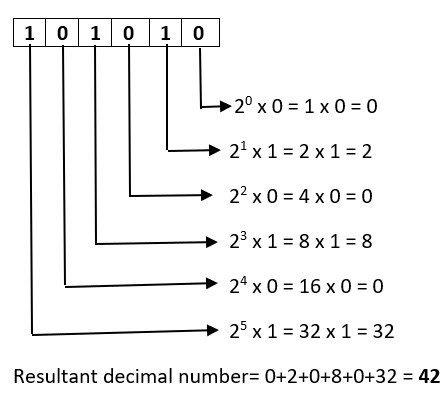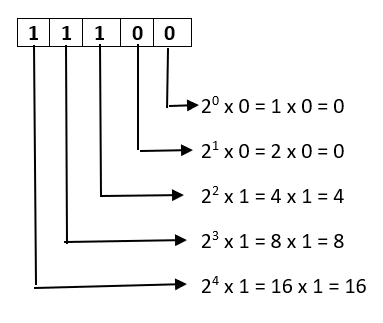Open in App
Not now

# How to Convert from Binary to Decimal?

• Last Updated : 29 Dec, 2021

Binary numbers are numbers that are understandable by computer machines. It is in a combination of 0’s & 1’s. As computers and few electronic devices understood only binary language they take input only in binary format and returns result in binary format.

In this article, we are going to learn how to convert a binary number to a decimal.

### Binary to Decimal Formula

To convert a binary number to decimal we need to perform a multiplication operation on each digit of a binary number from right to left with powers of 2 starting from 0 and add each result to get the decimal number of it.

This can be better explained using the below examples:

Example 1: Let’s consider a binary number 1111. We need to convert this binary number to a decimal number.

Solution:

As mentioned in the above paragraph while converting from binary to decimal we need to consider each digit in binary number from right to left.By this way, we can do binary to decimal conversion.

Note: We represent any binary number with this format (xxxx)2 and decimal in (xxxx)10 format.

Example 2: Convert (101010)2->(?)10

Solution:We keep on increasing the power of 2 as long as number of digits in binary number increases.

Example 3: Convert (11100)2->(?)10

Solution:Resultant Decimal number = 0+0+4+8+16 = 28

So (11100)2->(28)10

There is also another method called Doubling that can be used to convert binary numbers to decimals.

### Doubling Method

To explain this method we will consider an example and try to solve that stepwise.

Example 1: Convert Binary number (10001)2 to decimal.

Solution:

Similar to the above approach, In this approach also consider each digit but from left to right and performs step-wise computations on it.

Step-1 First we need to multiply 0 with 2 and add the 1st digit in binary number.

0 x 2 + 1 = 0 + 1 = 1

Step-2 Now use the result of above step and multiply with 2 and add the second digit of binary number.

1 x 2 + 0 = 2 + 0 = 2

The same step 2 is repeated until there will be no digit left. The final result will be the resultant decimal number.

2 x 2 + 0 = 4 + 0 = 4

4 x 2 + 0 = 8 + 0 = 8

8 x 2 + 1 = 16 + 1 = 17

So we performed step 2 on all remaining numbers and finally, we left with result 17 which is a decimal number for the given binary number.

So (10001)2->(17)10

Example 2: Convert (111)2 to decimal using doubling approach.

Solution:

0 x 2 + 1 = 0 + 1 = 1

1 x 2 + 1 = 2 + 1 = 3

3 x 2 + 1 = 6 + 1 = 7

The final result is 7 which is a Decimal number for 111 binary number. So (111)2->(7)10

These are the 2 approaches that can be used or applied to convert binary to decimal.

My Personal Notes arrow_drop_up